##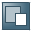Standard Functions### General

Values of elements or attributes of the XML message (the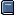payload ) are technically speaking a string. Therefore, all standard functions that operate on field values expect string arguments and return a string value. Nevertheless, the transferred value can of course have a different semantic data type, namely, the one that you specified when you defined the schema for the payload for the field. Standard functions exhibit the following standard behavior:

Depending on the standard function, data type conversions are used to ensure that the values are transferred in a format suitable for the function (using a cast). If the value cannot be interpreted, the mapping runtime triggers a Java exception.

If-clauses evaluate conditions that return Boolean values. Standard functions that return Boolean values return the string true or false. Standard functions that expect Boolean values interpret the values “1” and “true” (not case-sensitive) as true and all other values as false.### Overview of Functions

Data-flow objects for standard functions are structured as follows:

Most data-flow objects have two or three inbound channels on the left-hand side, and one outbound channel on the right-hand side.

If-functions are shaped as rhombuses or triangles.

There are also conversion functions with one inbound and outbound channel and functions that return a value without any input.

Standard functions that require additional specifications are indicated with a star (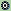). To call the dialog for Function Parameters, double-click the data-flow object.

The following figure provides an overview of all the different forms of data-flow objects: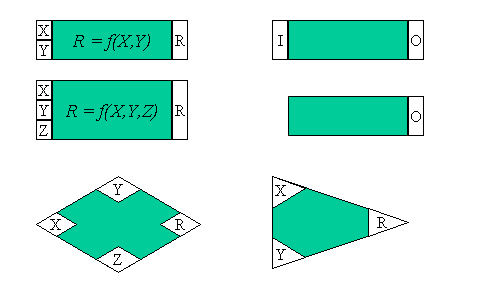To illustrate how the individual functions are used, the input boxes in the figure above have the variables X, Y, Z or I, and the result variable is either R or O. All standard functions in the tables below are described using these input and output variables. In the data-flow editor, other data-flow objects that are connected to the functions using the connecting boxes either return the input values or receive the result of the operation. You can combine the functions in any way you require (provided that the values transferred to a function can be interpreted).

#### Function Category: Arithmetic

You can only enter numerical values for this category (this includes values with digits after the decimal point). If the value cannot be interpreted as a digit, then the system triggers a Java exception. Otherwise, all calculations are executed with the precision of the Java data type float. The format of the result value depends on the result:

If the result is a value with digits after the decimal point, these remain unchanged.

Exception: If a zero follows the decimal point, then this is cut off. This means that the result of the calculation 4.2 0.2 is 4 and not 4.0.

Also note that Java values of type float are converted to the second system before the calculation. The result is calculated there and then this result is converted back to decimal format. The conversion may result in decimal places in the dyadic format that are periodically repeated and then automatically cut off. In the decimal system, this can result in inaccurate results (example: 2.11 + 22.11 = 24.220001). If the number of positions after the decimal point is to be restricted to two, for example, in the case of values for a currency, you can format the values after the calculation by using the standard function FormatNum.If the particular usage case requires more detailed results, you can also select the decimal system as the basis for the calculations. The Java class BigDecimal is used for internal purposes. For more details see SAP Note 958486.

 Function Name Function add R = X + Y subtract R = X - Y equalsA R = true, if value X equals value Y, otherwise R = false. The values are interpreted numerically, therefore the value 1.5 is the same as 1.50. abs O = Absolute value of I sqrt R is the square root of X sqr R is the square of X sign R = 1, when X is a positive number R = 0, when X equals 0 R = 1, when X is a negative number neg R = -X 1/x R is the reciprocal of X power R = XY less true, when X < Y, otherwise false greater true, when X > Y, otherwise false multiply R = X * Y divide R = X / Y max R = Maximum of values X and Y min R = Minimum of values X and Y ceil O = The smallest possible integer value (up to minus 'infinite’) that is not smaller than the argument I. Corresponds to the Java function java.lang.Math.ceil(). floor O = The largest possible integer value that is not larger than the argument I. Corresponds to the Java function java.lang.Math.floor(). round O = Integer value that is closest to the value of argument I. Corresponds to the Java function java.lang.Math.round(). counter O = Number of calls for this target-field mapping where you specify the initial value and the increment of the counter in the function parameters. FormatNum Converts I according to a pattern that you define using the function parameters. The possible patterns are the same as in the Java class java.text.DecimalFormat.

#### Function Category: Boolean

All functions in this category expect Boolean input values (see above).

 Function Name Function And R is true if X and Y have the value true. Otherwise, R is false. Or R is true if X or Y have the value true. Otherwise, R is false. Not ●      O is false if I has the value true ●      O is true if I has the value false Equals Compares both Boolean values X and Y and returns true if both are equal, otherwise false. Non-Boolean values are interpreted as false (see above). Use the functions equalsS or compare from the Text category to compare strings. notEquals R = Not(Equals(X,Y)) if ●      If condition X is fulfilled (returns true): R = Y ●      If condition X is not fulfilled (returns false): R = Z ifWithoutElse If condition X is satisfied (returns true): R = Y. Which Else value the function should return depends on various different factors (see detailed documentation).

#### Function Category: Constants

Since these functions do not have any input values, they are generating functions.

 Function Use Constant O gets any string constant that you can enter in the dialog for the function parameters. CopyValue For a frequently occurring element, copies the value at a defined position in the source structure to the assigned target field. sender O gets the name of the sender business system. Test_Sender_System is output when you test the message mapping in the Integration Builder. receiver O returns the name of the receiver business system. Test_Receiver_System is output when you test the message mapping in the Integration Builder.

#### Function Category: Conversions

 Function Use FixValues Executes a value mapping using a fixed value table that you complete using the function parameters. The table is saved together with the most current message mapping and can only be used once. Value mapping Executes a value mapping for the value I entered. In the function parameters, assign the both input value I and source value O to a representation in the value-mapping table. In this way, you can assign source and target structure fields to a representation. You complete the value-mapping table on the configuration maintenance screen in the Integration Builder. Since these entries are not available at design time, the mapping result is just an estimate when you test the message mapping. See alsoValue MappingThe runtime interface for value mappings has changed for XI 3.0. However, XI 2.0 message mappings that call the Value Mapping function still function using the old runtime interface. To use the function parameters in the new runtime interface, choose XI 3.0 Interface. In the function properties for the standard function Value mapping, in the fields Agency and Schema, enter the key for the source or target value. These values can have any values. However, you can also refer to the fields SenderParty, SenderService, ReceiverParty or ReceiverService. The mapping runtime replaces these values at runtime with the values from the corresponding fields in the message header of the message for which a value mapping is being executed.In the field Agency you can also enter the name of a business system. When transporting value mappings from one Integration Directory to another, the Integration Builder sees if you have entered a business system as the agency and converts it during the transport (see also:Configuring Groups and Transport Targets).

Function Category: Date

The following functions enable you to define the date format for the source or target format using the dialog for the function parameters.The format adheres to the convention as defined in the standard Java class java.util.SimpleDateFormat. The function parameters dialog provides you with a wizard that you can use to enter the most frequently used date formats.

 Function Use currentDate Returns the current date using O. This function is a generating function. DateTrans Converts date format I to another date format O. DateBefore R = true, when date X comes before date Y, otherwise false DateAfter R = true, when date X comes after date Y, otherwise false CompareDates R = 1, when date X is after date Y R = 0, when date X is the same as date Y R = -1, when date X is before date Y
##### Extended Settings

It is not only date formats that are country-specific; the count for weekdays and calendar weeks is also country-specific. You can specify the relevant conventions for these in the function parameters:

First Weekday
Specifies which day of the week is recorded as the first weekday. In the United States, for example, the first weekday is Sunday, while in France, it is Monday. The count begins at 1. The mapping runtime sets this attribute using the Java method

Minimum Number of Days Required in First Week
Specifies how many days the first week of the year must have for it to be considered the first calendar week. The mapping runtime sets this attribute using the Java method

Using the checkbox Calendar Is Lenient, you can control whether invalid dates trigger exceptions or not. If the checkbox is checked, the mapping runtime permits a date such as February 942, 1996, which is then interpreted as the 941st day after February 1, 1996. An exception is not triggered. The mapping runtime sets this attribute using the Java method setLenient(). For more information, see the Java documentation under http://java.sun.com/j2se/1.4.2/docs/api/java/util/Calendar.html#setLenient(boolean).

#### Function Category: Node Functions

 Function Use createIf If there are structuring elements in your target structure that do not exist in the source structure, insert them using this function. Using condition I you can control whether the element is inserted or not. You connect the element in the target structure using O. removeContexts Removes all higher-level contexts of a source field. In this way, you can delete all hierarchy levels and generate a list. replaceValue Replaces the value I with a value that you can define in the dialog for the function parameters. exists O = true, if the source field assigned to inbound channel I exists in the XML instance. Otherwise, false. SplitByValue Inserts a context change for an element. collapseContexts Deletes all values from all contexts from the inbound queue except for the first value. Empty contexts (= ResultList.SUPPRESS) are replaced by empty strings. Only one queue remains, which consists of contexts that contain just one value each. Finally, all internal context changes are deleted, so that all values belong to one and the same context. useOneAsMany Replicates a value of a field occurring once to pair it as a record with the values of a field occurring more than once. sort Sorts all values of the multiply-occurring inbound field I within the existing or set context. The sorting process is stable (the order of elements that are the same is not switched) and it sorts the values in O(n*log(n)) steps. Using the function parameters, you can specify whether values are to be sorted numerically or lexicographically (case-sensitive or non case-sensitive) and in ascending or descending order. sortByKey Like sort, but with two inbound parameters to sort (key/value) pairs. The sort process can be compared to that of a table with two columns. ●      Using the first parameter, you pass key values from the first column, which are used to sort the table. If you have classified the key values as numeric in the function parameters, they must not be equal to the constant ResultList.SUPPRESS. See also: The ResultList Object ●      Using the second parameter, you pass the values from the second column of the table. If there is a discrepancy between the number of keys and values, the mapping runtime triggers an exception. The function returns a queue with the values sorted according to the keys. mapWithDefault Replaces empty contexts in the inbound queue with a default value, which you specify in the function parameters. Example: If “Default” is the default value and A|B1,B2| |C| |D is the inbound queue, mapWithDefault returns the following outbound queue: A | B1,B2 | Default | C | Default |D. The function corresponds to the following combination of standard functions: If(   []field,   exists([]field),   Constant([value=default])) formatByExample This function has two inbound queues, which must both have the same number of values. To generate the result queue, the function takes the values from the first queue and combines them with the context changes from the second queue.

#### Function Category: Statistics

The functions of this function category are designed for source fields that occur more than once in the source structure (maxOccurs = i > 1).

 Function Use sum R = Sum of values X1 to Xi of a context. average R = Average of values X1 to Xi of a context count R = Number of fields in a context (i) index R = Index i of Xi. In the function parameters, you specify the following: the initial value of i, the increment, and whether the index value is to be reinitialized at the beginning of every new context, or whether it is to have the same value for the entire source structure.

#### Function Category: TextIn position specifications, the 0 position corresponds to the first character in the string.

 Function Use substring Returns a substring O for a string I. Use the dialog for the function properties to specify the position of the substring. Example: substring(“Hello”, 0,1) = “H”, means that the substring from Startindex 0 to Endindex 1 (not including position 1) is shown. concat R = Linking of strings X and Y (without blanks). Example: X = „Mrs.“; Y = „Miller“; R = „Mrs.Miller“. Use the dialog for the function properties to insert a separator in the string. equalsS R = true, if string X equals string Y, otherwise R = false. indexOf (2 input parameters) R = first position at which string Y is found in X and –1 if Y does not occur at all. indexOf (3 input parameters) R = first position from position Z at which string Y is found in X and –1 if Y does not occur at all. lastIndexOf (2 input parameters) R = last position at which string Y is found in X and –1 if Y does not occur at all. lastIndexOf (3 input parameters) R = last position from position z at which string Y is found in X and –1 if Y does not occur at all. compare Compares string X with string Y: R = 0, when the strings are equal R = positive number i, when X is lexicographically larger than Y R = negative number i, when X is lexicographically smaller than Y i specifies the difference between the two strings lexicographically. The function acts in the same way as the compareTo() method of the JDK class java.lang.String. replaceString X: String in which something is to be replaced Y: String to be replaced in X Z: String to replace Y R = String in which each occurrence of Y in X is replaced by Z.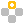X = "sparring with a purple porpoise" Y = “p” Z = “t” R = "starring with a turtle tortoise" length O = Length of string endsWith R = true when Y is the last string in X; otherwise false. startsWith (2 input parameters) R = true when Y is the first string in X; otherwise false. startWith (3 input parameters) R = true when Y matches X from position Z; otherwise false. toUpperCase Converts all lower case letters in I to upper case letters. trim Removes all white space characters (spaces, tabs, returns) at the start and end of a string. Acts the same as the trim() method of the JDK class java.lang.String. toLowerCase Converts all upper case letters in I to lower case letters.